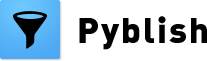# Pyblish DAG

I started taking notes about a potential implementation of Event Driven Processing (EDP) on Trello but figured I might as well share the adventure here by making a thread into a kind of journal.

If the goal of EDP is to allow for a graph-like structure of plug-ins, ordered arbitrarily and eventually visually as nodes and edges, then an alternative to EDP achieving the same goal is an directed acyclic graph (DAG), the practical difference being that one is dynamically discovering which task to perform next (EDP) whereas the other is static and decided upfront (DAG).

### Pros/Cons

The benefit of EDP may lie in it’s flexibility and potential for an infinitely large graph at the cost of not being able to map upfront what it is going to do, leading to behaviour that is difficult to debug and reproduce.

A DAG on the other hand is predetermined, meaning it is predictable, easy to reproduce and favours parallelism at the cost of requiring all dependencies to be available from the get-go.

Familiarity with implementing and programming with EDP (for me personally) comes from working with GUIs and Qt specifically, whereas the use and practice of DAGs comes from DCCs; Maya, Nuke and Houdini specifically.

### Implementation

After some research, it looks like DAG and Topological Sort are very common amongst task scheduling programs like Pyblish and relatively easy to implement (given you understand the basics).

I’m looking to have it implemented into Pyblish as an extension (pyblish-dag). Meaning it could potentially be a drop-in replacement for the `order` attribute currently in place to determine which plug-ins to run next.

Meaning you could potentially choose algorithm on a per-run basis, but more importantly means it can flourish (or perish) in isolation, making room for other algorithms including EDP.

Here’s a full implementation of a DAG along with Depth-First Search and Topological Sorting, the primary ingredients for setting up plug-in dependencies and ordering them accordingly, adapted from here.

``````import sys

class Graph(dict):
def __iter__(self):
for value in self.values():
yield value

newVertex = Vertex(key)
self[key] = newVertex
return newVertex

if f not in self:

if t not in self:

class Vertex(dict):
def __init__(self, id):
self.id = id
self.color = "white"
self.distance = sys.maxsize
self.pred = None
self.discovery = 0
self.finish = 0

def __iter__(self):
for value in self.values():
yield value

def __hash__(self):
return hash(self.id)

def __str__(self):
return str(self.id)

def __repr__(self):
return self.__str__()

@property
def connections(self):
return self.keys()

self[nbr] = weight

class DfsGraph(Graph):
def __init__(self):
self.time = 0

def dfs(self):
for vertex in self:
vertex.color = "white"
vertex.pred = -1
for vertex in self:
if vertex.color == "white":
self.visit(vertex)

def visit(self, start_vertex):
self.time += 1

start_vertex.color = "gray"
start_vertex.discovery = self.time
for next_vertex in start_vertex.connections:
if next_vertex.color == "white":
next_vertex.pred = start_vertex
self.visit(next_vertex)

start_vertex.color = "black"
start_vertex.finish = self.time

self.time += 1

def toposorted(g):
return sorted(g, key=lambda v: v.finish, reverse=True)

if __name__ == "__main__":
g = DfsGraph()

g.dfs() # Perform depth-first search
print toposorted(g)  # Return sorted vertices
# -> [1, 3, 2, 4, 5, 7, 6, 8]

``````

Where an `edge` represents a dependency (e.g. Validators before Extractors), a `Vertex` a plug-in and `Graph` their canvas in which they reside.

Here is the graph being built in ASCII.

``````      ___
| 1 |
|___|          # E.g. Selector
|
____|____
_|_       _|_
| 2 |     | 3 |
|___|     |___|     # E.g. Validators
|_________|
|
_|_
| 4 |
|___|          # E.g. Validator with custom order
|
_|_
| 5 |
|___|
|
____|____
|    |    |
_|_  _|_  _|_
| 6 || 7 || 8 |     # E.g. Extractors
|___||___||___|

``````

If you pay attention to the returned list of integers at the bottom of the implementation, you can see that the order makes sense. Here it is again.

``````# -> [1, 3, 2, 4, 5, 7, 6, 8]
``````

Like with the current method of sorting by order, the final order of plug-ins with the same value of `order` is undefined. But even here there are alternatives of various algorithms.

Examples from Wikipedia on Topological Sorting

• visual left-to-right, top-to-bottom
• smallest-numbered available vertex first
• fewest edges first
• largest-numbered available vertex first
• attempting top-to-bottom, left-to-right
• arbitrary

Each with it’s own pros/cons.

### UI/UX

With this in mind, from what I can tell, the difficult part then is how to draw it.

First off, graph drawing in itself is a vast topic. As can be seen by the shear number and size of available books. (Just Google “graph drawing”). The main focus of these is on the layout of a graph. I.e. finding an aesthetically pleasing configuration in which to put one node next to another such that it makes sense to a human being.

Once we’ve got the layout all figured out, the next task is then a choice of colors and shapes. Which of course is highly subjective, but we’ve got some good references out there in our daily lives, along with this collection of all visual programming languages out there.

Once that’s done, the next step is interactivity. How does one interact with these nodes? Also here we’ve got good reference and intuition since it’s part of our daily lives.

The final and possibly easiest step is then to map the visual portion of a graph into something we can feed into an algorithm like Topological Sort. As each Vertex can be anything, including a PyQt QWidget, this should be as easy as just giving each an instance variable `id` that the user adds to another QWidget by dragging from one to the other.

1 Like